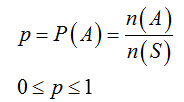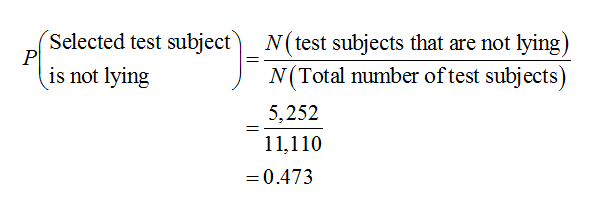# Refer to the sample data for polygraph tests shown below. If one of the test subjects is randomly​ selected, what is the probability that the subject is not​ lying? Is the result close to the probability of 0.4450.445for a negative test​ result? Did the Subject Actually Lie? No​ (Did Not​ Lie)Yes​ (Lied) Positive test results17174444Negative test results35351414The probability that a randomly selected polygraph test subject was not lying is nothing.​(Type an integer or decimal rounded to three decimal places as​ needed.)Is the result close to the​ probability, rounded to three decimal​ places, of 0.445for a negative test​ result?

Question
Refer to the sample data for polygraph tests shown below. If one of the test subjects is randomly selected, what is the probability that the subject is not lying? Is the result close to the probability of
0.4450.445
for a negative test result?

Did the Subject Actually Lie?
 No​ (Did Not​ Lie) Yes​ (Lied) 1717 4444 3535 1414
The probability that a randomly selected polygraph test subject was not lying is
nothing.
(Type an integer or decimal rounded to three decimal places as needed.)
Is the result close to the probability, rounded to three decimal places, of
0.445
for a negative test result?
check_circle

Step 1

Concept of probability:

Probability deals with the likelihood of occurrence of a given event. The probability value lies between 0 and 1. An event with probability 1 is considered as certain event and an event with probability 0 is considered as an impossible event. The probability of 0.5 infers of having equal odds of occurring and not occurring of an event.

The general formula to obtain probability of an event A is,

P(A) = (number of favourable elements for event A)/(Total number of elements in the sample space).

The basic properties of probability are given below:Step 2

Find the probability that the randomly selected polygraph test subject was not lying:

Given that from the sample data of polygraph tests, one test subject is randomly selected.

The total number of test subjects that are not lying is, the sum of test subjects that are not lying in positive results tests and negative results tests.

That is, the total number of test subjects that are not lying 1,717 + 3,535 = 5,252.

The total sample size is 1,717 + 3,535 + 4,444 + 1,414 = 11,110.

The probability that the randomly selected polygraph test subject was not lying is obtained as 0.473 from the calculation given below:Step 3

Check whether the probability that the randomly selected polygraph test subject was not lying is close to 0.445:

The probability that the randomly selected polygraph test subject was not lying is 0.47...

### Want to see the full answer?

See Solution

#### Want to see this answer and more?

Solutions are written by subject experts who are available 24/7. Questions are typically answered within 1 hour.*

See Solution
*Response times may vary by subject and question.
Tagged in

### Other